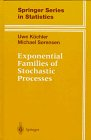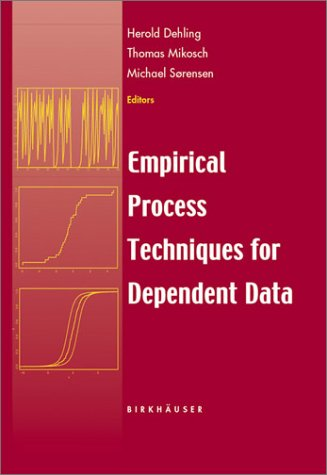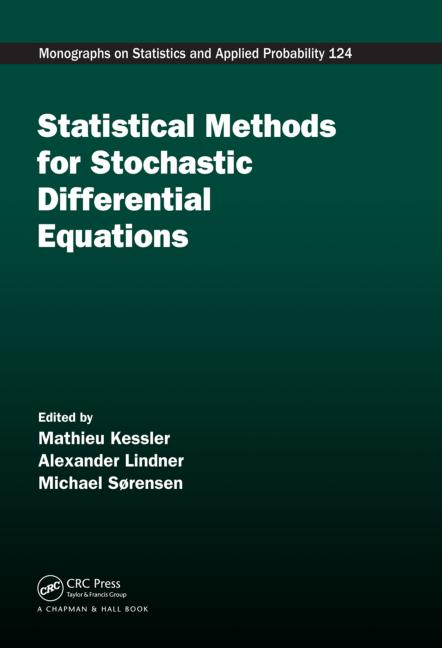# Michael Sørensen

Professor and Head of the Department of Mathematical Sciences, University of Copenhagen.

Here are some ways of contacting me:

E-mail: michael@math.ku.dk

Telephone (direct): +45 3533 0402
(dept.): +45 3532 0723/+45 3532 0724

Address: Department of Mathematical Sciences, University of Copenhagen, Universitetsparken 5, DK-2100 Copenhagen Ø, Denmark.

My main research interests are:

Statistical inference for stochastic processes, in particular discrete time sampling of continuous time processes such as models given by stochastic differential equations and jump processes. The interaction between statistics and finance. Stochastic models and related statistical problems, particularly in the physics of blown sand, turbulence and biology.

Full list of publications and CV:         List of publications       CV

Books:You can read about my work on exponential families of stochastic processes in my book Exponential Families of Stochastic Processes, co-authored with Uwe Küchler (Humboldt- University of Berlin).You may also be interested in the book Empirical Process Techniques for Dependent Data that I have edited jointly with Thomas Mikosch and Herold Dehling.Jointly with Mathieu Kessler and Alexander Lindner, I have edited the book Statistical Methods for Stochastic Differential Equations, where you can read about my work (and that of others) in this area. I have written the chapter on "Estimating functions for diffusion type processes".

Textbook in Danish:

The most comprehensive version of my introduction to probability theory for first year students is the 9th edition: En Introduktion til Sandsynlighedsregning.

Extended abstract collections:

Workshop on Stochastic Partial Differential Equations: Statistical Issues and Applications, co-edited with Marianne Huebner

Workshop on Dynamic Stochastic Modeling in Biology, co-edited with Marianne Huebner

Selected recent publications:

Estimating functions for jump-diffusions. Co-author: Nina Munkholt Jakobsen. Stoch. Proc. Appl., 129, 2019, 3282 - 3318.

Langevin diffusions on the torus: estimation and applications. Co-authors: Eduardo Garcia-Portugues, Kanti V. Mardi and Thomas Hamelryck. Statistics and Computing, 29, 2019, 1 - 22.

A review of asymptotic theory of estimating functions. Co-author: Jean Jacod Statistical Inference for Stochastic Processes, 21, 2018, 415 - 434.

A generative angular model of protein structure evolution Co-authors: Michael Golden, Eduardo Garcia-Portugues, Kanti V. Mardi, Thomas Hamelryck and Jotun Hein. Molecular Biology and Evolution, 34, 2017, 2085 - 2100.

Efficient estimation for diffusions sampled at high frequency over a fixed time interval. Co-author: Nina Munkholt Jakobsen. Bernoulli, 23, 2017, 1874 - 1910.

Simulation of multivariate diffusion bridges. Co-authors: Mogens Bladt and Samuel Finch. J. Roy. Statist. Soc. B, 78, 2016, 343 - 369.

Simple simulation of diffusion bridges with application to likelihood inference for diffusions. Co-author: Mogens Bladt. Bernoulli, 20, 2014, 645 - 675.

A transformation approach to modelling multi-modal diffusions. Co-author: Julie Lyng Forman. Journal of Statistical Planning and Inference, 146, 2014, 56 - 69.

Statistical inference for discrete-time samples from affine stochastic delay differential equations. Co-author: Uwe Küchler. Bernoulli, 19, 2013, 409 - 425.

Estimating functions for diffusion-type processes. In Kessler, M., Lindner, A. and Sørensen, M. (eds.): Statistical Methods for Stochastic Differential Equations, CRC Press - Chapmann and Hall, 2012, 1 - 107.

Prediction-based estimating functions: review and new developments. Brazilian Journal of Probability and Statistics, 25, 2011, 362 - 391.

Maximum likelihood estimation for integrated diffusion processes. Co-author: Fernando Baltazar-Larios. In Chiarella, C. and Novikov, A. (eds.): Contemporary Quantitative Finance: Essays in Honour of Eckhard Platen, Springer, Heidelberg, 2010, 407 - 423.

A simple estimator for discrete-time samples from affine stochastic delay differential equations. Co-author: Uwe Küchler. Statistical Inference for stochastic Processes, 13, 2010, 125 - 132.

Estimating functions for discretely sampled diffusion-type models. Co-authors: Bo Martin Bibby and Martin Jacobsen. In Ait-Sahalia, Y. and Hansen, L.P. (eds.): Handbook of Financial Econometrics, North Holland, Oxford, 2010, 203 - 268.

Unpublished papers:

Prediction-based estimation for diffusion models with high-frequency data

Co-authors: Emil S. Jørgensen

This paper obtains asymptotic results for parametric inference using prediction-based estimating functions when the data are high frequency observations of a diffusion process with an infinite time horizon. Specifically, the data are observations of a diffusion process at $n$ equidistant time points $\Delta_n i$, and the asymptotic scenario is $\Delta_n \to 0$ and $n\Delta_n \to \infty$. For a useful and tractable classes of prediction-based estimating functions, existence of a consistent estimator is proved under standard weak regularity conditions on the diffusion process and the estimating function. Asymptotic normality of the estimator is established under the additional rate condition $n\Delta_n^3 \to 0$. The prediction-based estimating functions are approximate martingale estimating functions to a smaller order than what has previously been studied, and new non-standard asymptotic theory is needed. A Monte Carlo method for calculating the asymptotic variance of the estimators is proposed.

Corrigendum to Simulation of multivariate diffusion bridges''

Co-authors: Mogens Bladt and Samuel Finch.

We correct an error in Theorem 1 in Bladt, Finch & Sørensen (2016), where the initial distribution of an auxiliary diffusion process that is used to describe the distribution of the proposed approximate diffusion bridge is wrong. As a consequence we also correct the pseudo marginal Metropolis-Hastings algorithm and the alternative MCMC-algorithm that have an exact diffusion bridge as their target distribution. The same auxiliary diffusion plays a central role in the two algorithms.

Corrigendum to Simple simulation of diffusion bridges with application to likelihood inference for diffusions''

Co-authors: Mogens Bladt and Marcin Mider.

We correct an error in Theorem 2.1 in Bladt & Sørensen (2014), where the initial distribution of an auxiliary diffusion process that is used to describe the distribution of the proposed approximate diffusion bridge is wrong. As a consequence, we also correct the pseudo marginal Metropolis-Hastings algorithm that has an exact diffusion bridge as its target distribution. The same auxiliary diffusion plays a central role in the algorithm.

OPTIMAL INFERENCE IN DYNAMIC MODELS WITH CONDITIONAL MOMENT RESTRICTIONS

Co-author: Bent Jesper Christensen

By an application of the theory of optimal estimating function, optimal instruments for dynamic models with conditional moment restrictions are derived. The general efficiency bound is provided, along with estimators attaining the bound. It is demonstrated that the optimal estimators are always at least as efficient as the traditional optimal generalized method of moments estimator, and usually more efficient. The form of our optimal instruments resembles that from Newey (1990), but involves conditioning on the history of the stochastic process. In the special case of i.i.d. observations, our optimal estimator reduces to Newey's. Specification and hypothesis testing in our framework are introduced. We derive the theory of optimal instruments and the associated asymptotic distribution theory for general cases including non-martingale estimating functions and general history dependence. Examples involving time-varying conditional volatility and stochastic volatility are offered.

EFFICIENT ESTIMATION FOR ERGODIC DIFFUSIONS SAMPLED AT HIGH FREQUENCY

A general theory of efficient estimation for ergodic diffusions sampled at high frequency is presented. High frequency sampling is now possible in many applications, in particular in finance. The theory is formulated in term of approximate martingale estimating functions and covers a large class of estimators including most of the previously proposed estimators for diffusion processes, for instance GMM-estimators and the maximum likelihood estimator. Simple conditions are given that ensure rate optimality, where estimators of parameters in the diffusion coefficient converge faster than estimators of parameters in the drift coefficient, and for efficiency. The conditions turn out to be equal to those implying small $\Delta$-optimality in the sense of Jacobsen and thus gives an interpretation of this concept in terms of classical statistical concepts. Optimal martingale estimating functions in the sense of Godambe and Heyde are shown to be give rate optimal and efficient estimators under weak conditions.

SMALL DISPERSION ASYMPTOTICS FOR DIFFUSION MARTINGALE ESTIMATING FUNCTIONS

Martingale estimating functions provide a flexible and powerful framework for statistical inference about diffusion models based on discrete time observations. We supplement the standard results on large sample asymptotics by results on small dispersion asymptotics, which can be applied in situations where the noise term is sufficiently small, compared to the drift term, that a Gaussian approximation to the diffusion can be used. The theory, which is based on the stochastic Taylor expansion, covers proper likelihood inference too. It is remarkable that the martingale property of an estimating function also for small dispersion asymptotics ensures that estimators are consistent. A model from mathematical finance is considered in detail. For this example the range of applicability of the small dispersion asymptotics is investigated in a simulation study of the distribution of estimators.

EXPONENTIAL FAMILY INFERENCE FOR DIFFUSION MODELS

We consider ergodic diffusion processes for which the class of invariant measures is an exponential family, and study inference based on the class of invariant probability measures when the diffusion has been observed at discrete time points. When the drift depends linearly on the parameters, the invariant measures form an exponential family. It is investigated how the usual exponential family inference, which can be done by means of standard statistical computer packages, works when the observations are from a diffusion process. In particular, the limit distributions of estimators and test statistics are derived. As an example, we consider classes of diffusions with generalized inverse Gaussian marginals. A particular instance is the well-known Cox-Ingersoll-Ross model from mathematical finance.

ON THE MOMENTS OF SOME FIRST PASSAGE TIMES FOR EXPONENTIAL FAMILIES OF PROCESSES

For curved exponential families of stochastic processes a natural and often studied sequential procedure is to stop observation when a linear combination of the coordinates of the canonical process crosses a prescribed level. Conditions are given which ensure that such first passage times, or a function of them, have finite moments. Also results about L_p convergence as the prescribed level tends to infinity are given.

Preprints:

J. Møller and M. Sørensen: Parametric models of spatial birth-and-death processes with a view to modelling linear dune fields
Research Report No. 184, Department of Theoretical Statistics, University of Aarhus, 1990.

This homepage was last updated on July 28, 2020.